# SAT II Chemistry : pH

## Example Questions

### Example Question #2 : Reactions And Equilibrium

A scientist makes a solution by adding 0.2 grams of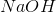to enough water so that the resulting solution has a volume of 10 liters. What, approximately, is the pH of this solution?has a molar mass of approximately 40 g/mol, meaning that there is 0.01 mol of it in the solution. Sodium hydroxide is a strong base and completely dissociates in water. Its concentration in the solution is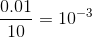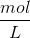. This means that the concentration of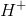ions is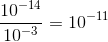and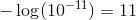. Thus, the pH of the solution is 11.